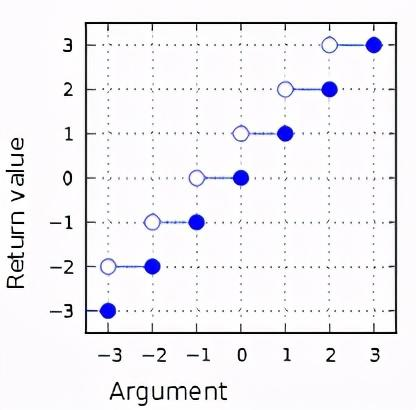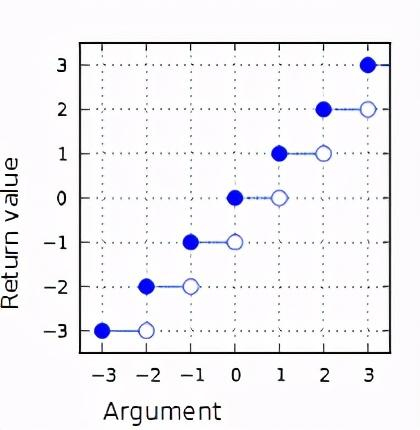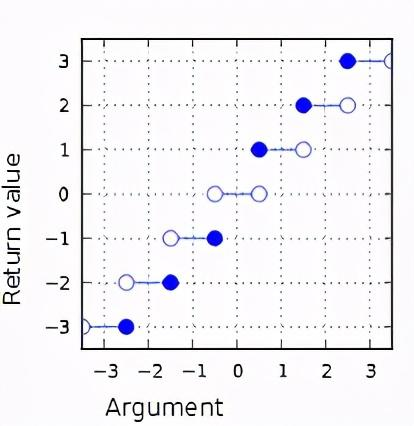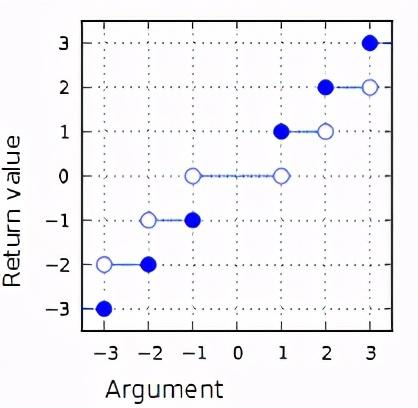ceil函数：向上取整floor函数：向下取整round函数：四舍五入取整trunc函数：舍尾取整

ceil函数

ceil函数如下定义，函数返回不小于参数arg的最小整数，即向上取整。

`float ceil ( float arg );double ceil ( double arg );`ceil函数

`#include <cmath>#include <iostream>int main(){    // ceil    std::cout << "ceil(+3.3) = " << std::ceil(+3.3) << '\n'              << "ceil(-3.4) = " << std::ceil(-3.4) << '\n'              << "ceil(-0.0) = " << std::ceil(-0.0) << '\n'              << "ceil(-Inf) = " << std::ceil(-INFINITY) << '\n';}`

`ceil(+3.3) = 4ceil(-3.4) = -3ceil(-0.0) = -0ceil(-Inf) = -inf`

floor函数

floor函数如下定义，函数返回不大于arg的最大整数值，即向下取整。

`float floor ( float arg );double floor ( double arg );`floor函数

`#include <cmath>#include <iostream>int main(){    // floor    std::cout << "floor(+3.6) = " << std::floor(+3.6) << '\n'              << "floor(-3.2) = " << std::floor(-3.2) << '\n'              << "floor(-0.0) = " << std::floor(-0.0) << '\n'              << "floor(-Inf) = " << std::floor(-INFINITY) << '\n';}`

`floor(+3.6) = 3floor(-3.2) = -4floor(-0.0) = -0floor(-Inf) = -inf`

round函数

round函数是我们最常用的取整方式，通过四舍五入方式取整，其定义如下：

`float round ( float arg );double round ( double arg );`round

`#include <iostream>#include <cmath>int main(){    // round    std::cout << "round(+4.2) = " << std::round(4.2)  << '\n'              << "round(+4.5) = " << std::round(4.5)  << '\n'              << "round(+4.7) = " << std::round(4.7)  << '\n'              << "round(-4.3) = " << std::round(-4.3) << '\n'              << "round(-4.5) = " << std::round(-4.5) << '\n'              << "round(-4.8) = " << std::round(-4.8) << '\n'; }`

`round(+4.2) = 4round(+4.5) = 5round(+4.7) = 5round(-4.3) = -4round(-4.5) = -5round(-4.8) = -5`

trunc函数

`float trunc ( float arg );double trunc ( double arg );`trunc函数

`#include <cmath>#include <iostream>int main(){    // trunc    std::cout << "trunc(+2.7) = " << std::trunc(+2.7) << '\n'              << "trunc(-2.9) = " << std::trunc(-2.9) << '\n'              << "trunc(-0.0) = " << std::trunc(-0.0) << '\n'              << "trunc(-Inf) = " << std::trunc(-INFINITY) << '\n';}`

`trunc(+2.7) = 2trunc(-2.9) = -2trunc(-0.0) = -0trunc(-Inf) = -inf`

-- End --